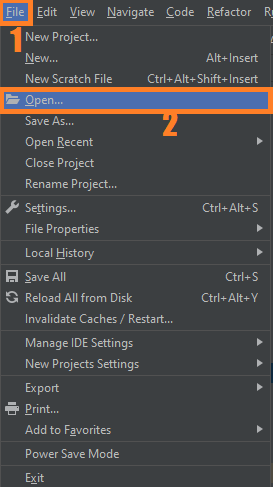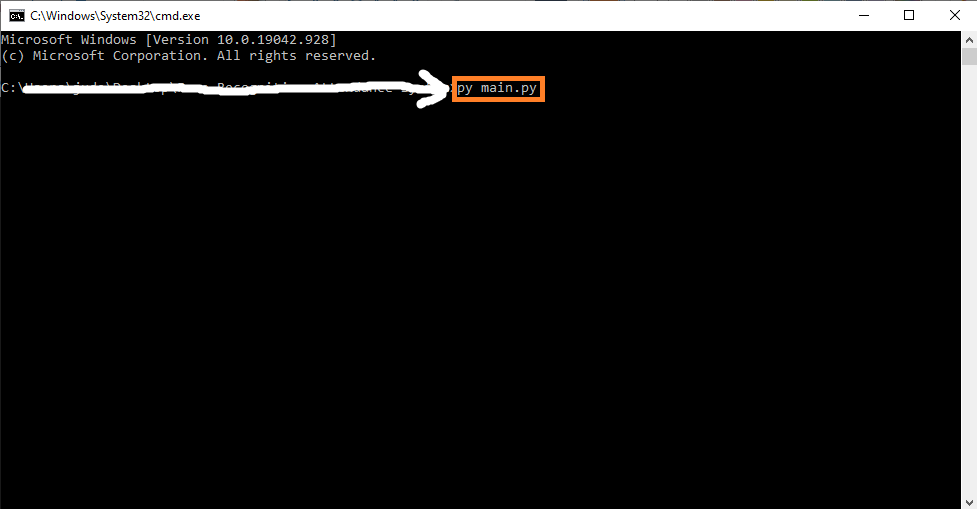# Image Blending OpenCV Python With Source Code

## Image Blending OpenCV Python With Source Code

The Image Blending OpenCV Python was developed using Python OpenCV, This Project is Mixing up of two images. In this Article we will learn how image Blending works in OpenCV Python.

There are many designing tools out there, but our goal is to create a beautiful design without using any of those software in this project. After working on this project, you will also have an idea on how to work with the OpenCV package. It is a great skill to have, especially if you like computer vision. You can follow a similar structure when working on similar OpenCV projects.

## What is OpenCV?

OpenCV (Open Source Computer Vision Library) is an open-source computer vision and machine learning software library. OpenCV was built to provide a common infrastructure for computer vision applications and to accelerate the use of machine perception in commercial products.

By the way if you are new to python programming and you don’t know what would be the the Python IDE to use, I have here a list of Best Python IDE for Windows, Linux, Mac OS that will suit for you. I also have here How to Download and Install Latest Version of Python on Windows.

To start executing Image Blending OpenCV Python With Source Code, make sure that you have installed Python 3.9 and PyCharm on your computer.

## Image Blending OpenCV Python With Source Code : Steps on how to run the project

Time needed: 5 minutes.

These are the steps on how to run Image Blending OpenCV Python With Source Code

First, download the given source code below and unzip the source code.• Step 2: Import the project to your PyCharm IDE.• Step 3: Run the project.

last, run the project with the command “py main.py”## Installed Libraries

```import cv2 as cv
import numpy as np
from matplotlib import pyplot as plt```

## Complete Source Code

```import cv2 as cv
import numpy as np
from matplotlib import pyplot as plt

def blend():
'''
Image Blending Using Pyramid
2. Find Gaussian Pyramids for two images. Upto 6 level
3. From Gaussian Pyramids,find their Laplacian Pyramids.
4. Now Join the images in each levels of laplacian P.
5. Finally from this joint image Pyramids, reconstruct the
Original Image.
'''

print(n1.shape)
print(n2.shape)

# n1_n2 = np.hstack((n1[:, :256], n2[:, 256:]))

'''
Generate Gaussian Pyramid for Nature 1
'''
n1_copy = n1.copy()
gp_n1 = [n1_copy]

for i in range(6):
n1_copy = cv.pyrDown(n1_copy)
gp_n1.append(n1_copy)

'''
Generate Gaussian Pyramid for Nature 2
'''
n2_copy = n2.copy()
gp_n2 = [n2_copy]

for i in range(6):
n2_copy = cv.pyrDown(n2_copy)
gp_n2.append(n2_copy)

'''
Laplacian Pyramid for Nature 1
'''
n1_copy = gp_n1
lp_n1 = [n1_copy]

for i in range(5, 0, -1):
gp_ex = cv.pyrUp(gp_n1[i])
lap = cv.subtract(gp_n1[i-1], gp_ex)
lp_n1.append(lap)

'''
Laplacian Pyramid for Nature 2
'''
n2_copy = gp_n2
lp_n2 = [n2_copy]

for i in range(5, 0, -1):
gp_ex = cv.pyrUp(gp_n2[i])
lap = cv.subtract(gp_n2[i-1], gp_ex)
lp_n2.append(lap)

'''
Join Half the Pyramid
'''
n1_n2_pyramid = []
n = 0
for n1_lap, n2_lap in zip(lp_n1, lp_n2):
n += 1
cols, rows, ch = n1_lap.shape
laplacian = np.hstack(
(n1_lap[:, 0:int(cols/2)], n2_lap[:, int(cols/2):]))
n1_n2_pyramid.append(laplacian)

'''
Reconstruct
'''
reconstruct = n1_n2_pyramid
for i in range(1, 6):
reconstruct = cv.pyrUp(reconstruct)

# cv.imshow("Nature 1", n1)
# cv.imshow("Nature 2", n2)
cv.imshow("N1_N2", reconstruct)
cv.waitKey()
cv.destroyAllWindows()

if __name__ == "__main__":
blend()
```